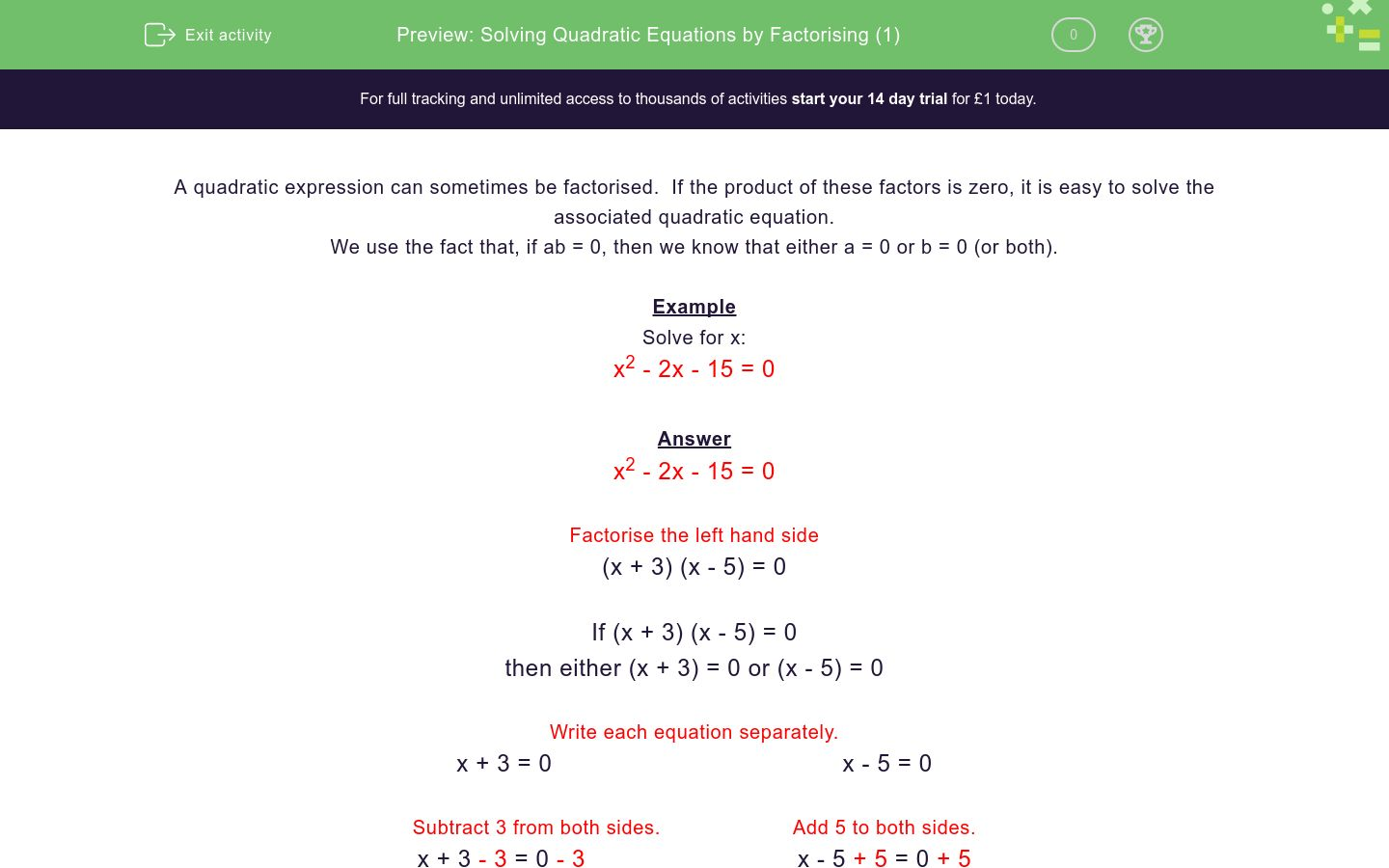# Solving Quadratic Equations by Factorising (1)

In this worksheet, students solve quadratic equations by factorising.Key stage:  KS 4

Curriculum topic:  Algebra

Curriculum subtopic:  Solve Quadratic Equations by Factorising

Difficulty level:### QUESTION 1 of 10

A quadratic expression can sometimes be factorised.  If the product of these factors is zero, it is easy to solve the associated quadratic equation.

We use the fact that, if ab = 0, then we know that either a = 0 or b = 0 (or both).

Example

Solve for x:

x2 - 2x - 15 = 0

Answer

x2 - 2x - 15 = 0

Factorise the left hand side

(x + 3) (x - 5) = 0

If (x + 3) (x - 5) = 0

then either (x + 3) = 0 or (x - 5) = 0

Write each equation separately.

x + 3 = 0                                         x - 5 = 0

Subtract 3 from both sides.                      Add 5 to both sides.

x + 3 - 3 = 0 - 3                              x - 5 + 5 = 0 + 5

Simplify.

x = -3                                             x = 5

Solution is:

x = -3 or x = 5

Solve the quadratic equation by selecting all the correct solutions below.

x2 - 3x - 18 = 0

x = 3

x = -3

x = -6

x = 6

Solve the quadratic equation by selecting all the correct solutions below.

x2 - x - 12 = 0

x = 4

x = -4

x = -3

x = 3

Solve the quadratic equation by selecting all the correct solutions below.

x2 + 11x + 28 = 0

x = -7

x = 7

x = 4

x = -4

Solve the quadratic equation by selecting all the correct solutions below.

x2 + x  = 0

x = ½

x = -1

x = 1

x = 0

Solve the quadratic equation by selecting all the correct solutions below.

x2 + 7x - 8 = 0

x = 8

x = -1

x = -8

x = 1

Solve the quadratic equation by selecting all the correct solutions below.

x2 + 8x + 16 = 0

x = 4

x = -4

x = -8

x = 16

Solve the quadratic equation by selecting all the correct solutions below.

x2 - 11x +30 = 0

x = 6

x = -6

x = -5

x = 5

Solve the quadratic equation by selecting all the correct solutions below.

x2 + 2x - 15 = 0

x = -5

x = 5

x = 3

x = -3

Solve the quadratic equation by selecting all the correct solutions below.

x2 - 3x - 28 = 0

x = -7

x = 4

x = 7

x = -4

Solve the quadratic equation by selecting all the correct solutions below.

x2 - 90x - 1000 = 0

x = -100

x = -10

x = 10

x = 100

• Question 1

Solve the quadratic equation by selecting all the correct solutions below.

x2 - 3x - 18 = 0

CORRECT ANSWER
x = -3
x = 6
EDDIE SAYS
(x-6)(x+3)=0
• Question 2

Solve the quadratic equation by selecting all the correct solutions below.

x2 - x - 12 = 0

CORRECT ANSWER
x = 4
x = -3
EDDIE SAYS
(x-4)(x+3)=0
• Question 3

Solve the quadratic equation by selecting all the correct solutions below.

x2 + 11x + 28 = 0

CORRECT ANSWER
x = -7
x = -4
EDDIE SAYS
(x+7)(x+4)=0
• Question 4

Solve the quadratic equation by selecting all the correct solutions below.

x2 + x  = 0

CORRECT ANSWER
x = -1
x = 0
EDDIE SAYS
x(x+1)=0
• Question 5

Solve the quadratic equation by selecting all the correct solutions below.

x2 + 7x - 8 = 0

CORRECT ANSWER
x = -8
x = 1
EDDIE SAYS
(x-1)(x+8)=0
• Question 6

Solve the quadratic equation by selecting all the correct solutions below.

x2 + 8x + 16 = 0

CORRECT ANSWER
x = -4
EDDIE SAYS
(x+4)(x+4)=0
• Question 7

Solve the quadratic equation by selecting all the correct solutions below.

x2 - 11x +30 = 0

CORRECT ANSWER
x = 6
x = 5
EDDIE SAYS
(x-6)(x-5)=0
• Question 8

Solve the quadratic equation by selecting all the correct solutions below.

x2 + 2x - 15 = 0

CORRECT ANSWER
x = -5
x = 3
EDDIE SAYS
(x-3)(x+5)=0
• Question 9

Solve the quadratic equation by selecting all the correct solutions below.

x2 - 3x - 28 = 0

CORRECT ANSWER
x = 7
x = -4
EDDIE SAYS
(x-7)(x+4)=0
• Question 10

Solve the quadratic equation by selecting all the correct solutions below.

x2 - 90x - 1000 = 0

CORRECT ANSWER
x = -10
x = 100
EDDIE SAYS
(x-100)(x+10)=0
---- OR ----

Sign up for a £1 trial so you can track and measure your child's progress on this activity.

### What is EdPlace?

We're your National Curriculum aligned online education content provider helping each child succeed in English, maths and science from year 1 to GCSE. With an EdPlace account you’ll be able to track and measure progress, helping each child achieve their best. We build confidence and attainment by personalising each child’s learning at a level that suits them.

Start your £1 trial